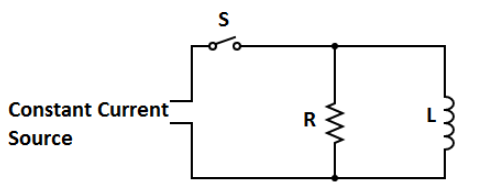Courses
Courses for Kids
Free study material
Offline Centres
MoreLast updated date: 04th Dec 2023
Total views: 279.3k
Views today: 4.79k

# In the given figure, after switch $S$ is closed at time $t = 0$, the emf of the source is automatically adjusted to maintain a constant current i through S. (a) Find the current through the inductor as a function of time.(b) At what time is the current through the resistor equal to the current in the inductor?Verified
279.3k+ views
Hint: In the figure, we have a Resistor and inductor in the circuit and the current flowing through LR circuit is given as $i = {i_0}(1 - {e^{\dfrac{{ - Rt}}{L}}})$ where ${i_0} = \dfrac{E}{R}$ which is called maximum current of the circuit, Junction rules we will find current in the inductor as a function of time.

(a) Let us suppose the current ${i_1}$ flows in the resistor in downward direction and current ${i_2}$ flows in the inductor in downward direction when the switch is closed at $t = 0$. Total current through Current source says $i$.Then we have,
$i = {i_1} + {i_2}$
Now, the voltages across resistor is $R{i_1}$ and voltage across inductor is given by,
$- L\dfrac{{d{i_2}}}{{dt}}$
So, $R{i_1} - L\dfrac{{d{i_2}}}{{dt}} = 0$
$L\dfrac{{d{i_1}}}{{dt}} + {i_1}R = 0$
Above equation is similar to the general equation $E = L\dfrac{{di}}{{dt}} + Ri$
Whose solution is given by $i = {i_0}{e^{\dfrac{{ - Rt}}{L}}}$
So we get the solution as,
${i_1} = i{e^{\dfrac{{ - Rt}}{L}}}$
Since, at $t = 0$ ${i_1} = 0$ and maximum current is ${i_0} = i$
So we get,
${i_2} = i - {i_1}$
$\therefore {i_2} = i(1 - {e^{\dfrac{{ - Rt}}{L}}})$ Which is the current through the inductor.

Hence, current through the inductor is given by ${i_2} = i(1 - {e^{\dfrac{{ - Rt}}{L}}})$.

(b) Since from part (i) we have,
${i_1}= i{e^{\dfrac{{ - Rt}}{L}}}$
$\Rightarrow {i_2} = i(1 - {e^{\dfrac{{ - Rt}}{L}}})$
Equate both equations we get,
$(1 - {e^{\dfrac{{ - Rt}}{L}}}) = {e^{\dfrac{{ - Rt}}{L}}}$
Taking Natural logarithm on both sides:
$(\dfrac{{Rt}}{L}) = \ln 2$
$\therefore t = \dfrac{L}{R}\ln 2$

Hence, the time at which current through inductor and resistor is $t = \dfrac{L}{R}\ln 2$.

Note: Remember, when the switch is just closed the charges start to flow in the circuit as circuit gets completed and the inductor starts to build a magnetic field around it as the inductor reaches the maximum point of producing magnetic field it acts as a short circuit.The time at which inductor is fully charged is known as its time constant.